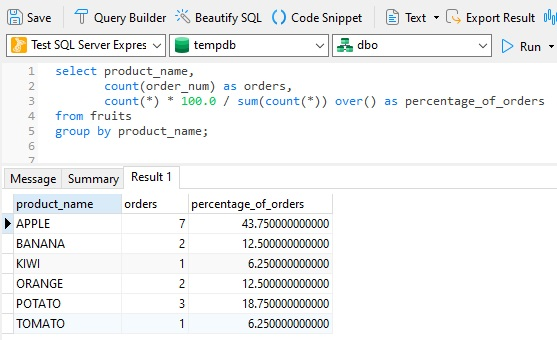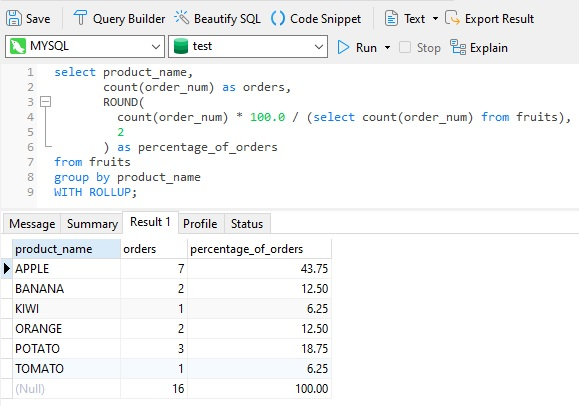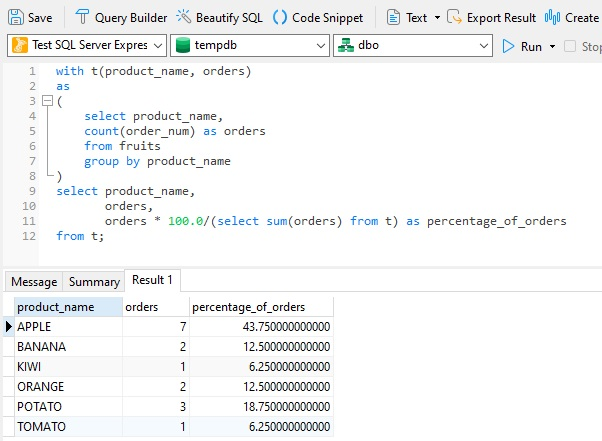# Navicat Blog

Calculating Percentage of Total Rows In SQL Jan 14, 2022 by Robert Gravelle

There are many times where you'll want to see the relative contribution of a row (or group of rows) to the total row count. In other words, what percentage of the total count a row represents. To illustrate, let's take the following table, shown in Navicat Premium 16:We can easily find out how many orders were received for each type of fruit by combining the count() function with the Group By clause:So now, how would we view what percentage each fruit's orders contributed to the total number of orders? In fact, there are three standard ways to calculate row percentages in SQL. They are:

• Using OVER() clause
• Using subquery
• Using a Common Table Expression, or CTE

The rest of this blog will explore each of these in turn.

# The OVER() Clause

Used predominantly with Window Functions, the OVER clause is used to determine which rows from the query are applied to the function, what order they are evaluated in by that function, and when the function's calculations should restart.

The OVER clause is the most efficient way to calculate row percentages in SQL, so it should be your first choice if efficiency is a priority for you. Here's the formula to obtain a percentage:

```count(*) * 100.0 / sum(count(*)) over()
```

Adding the above SQL to our original query produces the following results:Looks good, but some rounding wouldn't hurt. Unfortunately, that's not easily done using the over() clause. Perhaps the next option will be more to your liking.

# Using a Subquery

Not all databases support the OVER() clause, so the subquery approach can be a very valuable fallback solution. It's sometimes referred to as the "universal solution" since it works in all databases. Another benefit of this approach is that it is also the easiest to incorporate with functions such as Round(). Here is what we'll need to add to our query:

```count(*) * 100.0 / (select count(*) from <YourTable>)
```

And here is the universal solution in action:# Using a Common Table Expression (CTE)

The With common_table_expression clause specifies a temporary named result set, known as a common table expression (CTE). We can then select from the temporary result set to apply more functions to retrieved fields. IN our case, we can apply the sum() function to the counts to obtain the percentages:Keep in mind that this approach is the least efficient as the CTE basically runs a second query against the results of the inner (initial) one. That being said, there may be times that you'll need to use a CTE to perform additional processing that you couldn't easily do in one go.

# Conclusion

In this blog, we learned three ways to express the relative contribution of a row (or group of rows) to the total row count as a percentage. Each approach has its own strengths and weaknesses, so you'll have to choose one based on your specific requirements.

If you'd like to give Navicat 16 a try, you can download a 14-day fully functional FREE trial of Navicat here.

Blog Archives
Share
• Key Topics•• Account••• Others